International
Tables for
Crystallography
Volume C
Mathematical, physical and chemical tables
Edited by E. Prince

International Tables for Crystallography (2006). Vol. C, ch. 8.5, pp. 707-708

## Section 8.5.2. Lack of fit

E. Princea and C. H. Spiegelmanb

aNIST Center for Neutron Research, National Institute of Standards and Technology, Gaithersburg, MD 20899, USA, and bDepartment of Statistics, Texas A&M University, College Station, TX 77843, USA

### 8.5.2. Lack of fit

| top | pdf |

We saw in Section 8.4.2that the sum of squared residuals from an ideally weighted, least-squares fit to a correct model is a sum of terms that has expected value (np) and is distributed as χ2 with ν = np degrees of freedom. Further, the residuals have a distribution with zero mean. A value for the sum that exceeds (np) by an amount that is improbably large is an indication of lack of fit, which may be due to an incorrect model for the mean or to nonideal weighting or both. {The sum, S, may be considered to be improbably large when the value of the χ2 cumulative distribution function,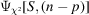, is close to 1.0. A value for the sum that is substantially less than (np) may also be an indication that the model contains more parameters than can be justified by the data set. Note also that a reasonable value for the sum of squared residuals does not prove that the model is correct. It indicates that the model adequately describes the data, but it in no way rules out the existence of alternative models that describe the data equally well.} If the sum of squares is greater than (np), it is commonly assumed that the mean model is correct, and that the weights have appropriate relative values, although their absolute values may be too large. If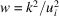, where k is some number greater than one, and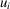is the standard uncertainty of the ith observation, the goodness-of-fit parameter,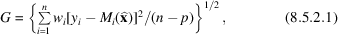is taken to be an estimate of k, and all elements of the inverse of the normal-equations matrix are multiplied by k2 to obtain the estimated variance–covariance matrix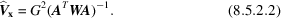Frequently, however, there is some other, independent estimate of the variance of the observation,, derived, for example, from counting statistics or from the observed scatter among symmetry-equivalent reflections. If this estimate is inconsistent with the hypothesis that all data points have been overweighted by a constant factor, then the assumption that the parameter estimates are unbiased but less precise than the original weights would indicate must be discarded. Instead, it must be assumed that the model is incorrect, or at least incomplete. A systematic error may be considered to cause the model to be incomplete, and may introduce bias into some or all of the refined parameters. (Note that in many standard statistical texts it is implicitly assumed, without so stating, that the data have already been scaled by a set of correct, relative weights. It is thus easy for the unwary reader to make the error of assuming that the practice of multiplying by the goodness-of-fit parameter is a well established procedure.)

The use of (8.5.2.2)to compute estimated variances and standard uncertainties assumes implicitly that the effect of lack of fit on parameter estimates is random, and applies equally to all parameters, even though different types of parameter may have very different mathematical relations in the model. With a model as complex as the crystallographic structure-factor formula, this assumption is certainly questionable.

Information about the nature of the model inadequacies can be obtained by examining the residuals (Belsley, Kuh & Welsch, 1980; Belsley, 1991). The standardized residuals,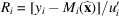, whereis the least-squares estimate of the parameters, should be randomly distributed, with zero mean, not only for the data set as a whole but also for subsets of the data that are chosen in a manner that depends only on the model and not on the observed values of the data. Here,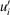is the standard uncertainty of the residual and is related to, the standard uncertainty of the observation, by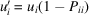, whereis a diagonal element of the projection matrix (Section 8.4.4). A scatter plot, in which the residuals are plotted against some control variable, such as |Fcalc|,, or one of the Miller indices, should reveal no general trends. The existence of any such trend may indicate a systematic effect that depends on the corresponding variable. The model may then be modified by inclusion of a factor that is proportional to that variable, and the refinement repeated. An examination of the shifts in the other parameters, and of the new row or column of the variance–covariance matrix, will then reveal which of the parameters in the unmodified model are likely to have been biased by the systematic effect. When this procedure has been followed, it is extremely important to consider carefully the nature of the additional effect and determine whether it is plausible in terms of physics and chemistry.

Another procedure for detecting systematic lack of fit makes use of the fact that, if the model is correct, and the error distribution is approximately normal, or Gaussian, the distribution of residuals will also be approximately normal. A large sample may be checked for normality by means of a quantile–quantile, or QQ, plot (Abrahams & Keve, 1971; Kafadar & Spiegelman, 1986). To make such a plot, the residuals are first sorted in ascending order of magnitude. If there are n points in the data set, the value of the ith sorted residual should be close to the value, xi, for which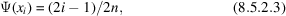where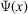is the cumulative distribution function for the normal p.d.f. A plot of Ri against xi should be a straight line with zero intercept and unit slope. A straight line with a slope greater than one suggests that the model is satisfactory, but that the variances of the data points have been systematically underestimated. Lack of fit is suggested if the curve has a higher slope near the ends, indicating that large residuals occur with greater frequency than would be predicted by the normal p.d.f.

The sorted residuals tend to be strongly correlated. A positive displacement from a smooth curve tends to be followed by another positive displacement, and a negative one by another negative one, which gives the QQ plot a wavy appearance, and it may be difficult to decide whether it is a straight line or not. Because of this, a useful alternative to the QQ plot is the conditional QQ plot (Kafadar & Spiegelman, 1986), so called because the abscissa for plotting the ith sorted residual is the mean of a conditional p.d.f. for that residual given the observed values of all the others. To construct a conditional QQ plot, first transform the distribution to a uniform p.d.f. by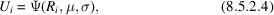where μ and σ are resistant estimates (Section 8.2.2) of the mean and standard deviation of the p.d.f., such as the median and 0.75 times the interquartile range, and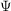represents the cumulative distribution function. Letting U0 = 0 and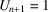, the expected value of Ui, given all the others, is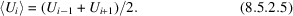The ith abscissa for the QQ plot is then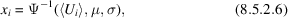where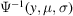is a per cent point function, or p.p.f., the value of x for which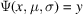.

QQ plots for subsets of the data can reveal, by nonzero intercepts, that those subsets are subject to a systematic bias. Because of its property of removing short-range kinks in the curve, the conditional QQ plot can be particularly useful in this application. The values of μ and σ used for the transformation to a uniform distribution, as in (8.5.2.4), should be those determined from the entire data set.

A QQ plot will reveal data points that are in poor agreement with the model, but that do not belong to any easily identifiable subset. Because of the central limit theorem (Section 8.4.1), however, the least-squares method tends to force the distribution of the residuals toward a normal distribution, and the discrepant points may not be clearly evident. A robust/resistant procedure (see Section 8.2.2), because it reduces the influence of strongly discrepant data points, helps to separate them from the body of the data. Therefore, if a data set contains discrepant points, a QQ plot of the residuals from a robust/resistant fit will tend to have greater curvature at the extremes than one from a corresponding least-squares fit. If the discrepant data points that are thus identified have a pattern, this information may enable a systematic error to be characterized.

### References

Abrahams, S. C. & Keve, E. T. (1971). Normal probability plot analysis of error in measured and derived quantities and standard deviations. Acta Cryst. A27, 157–165.
Belsley, D. A. (1991). Conditioning diagnostics. New York: John Wiley & Sons.
Belsley, D. A., Kuh, E. & Welsch, R. E. (1980). Regression diagnostics. New York: John Wiley & Sons.
Kafadar, K. & Spiegelman, C. H. (1986). An alternative to ordinary Q–Q plots: conditional Q–Q plots. Comput. Stat. Data Anal. 4, 167–184.